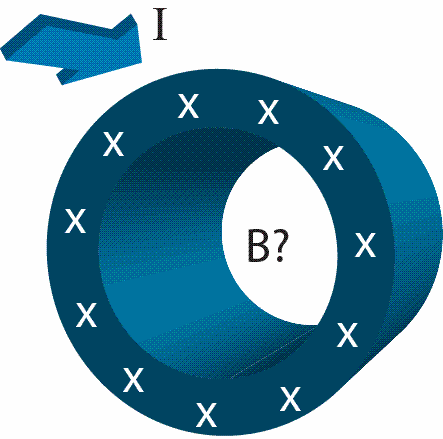# Problem: What is the direction of the magnetic field inside the tube?a. clockwiseb. counterclockwisec. radially inward to the centerd. radially outward from the centere. the magnetic field is zero

###### FREE Expert Solution

The tube is equivalent to a straight conductor. The magnetic field around a conductor is given by Amperes law.

Amperes law:

$\overline{)\begin{array}{rcl}\mathbf{\oint }\mathbf{B}\mathbf{·}\mathbf{d}\mathbf{L}& {\mathbf{=}}& {\mathbf{\mu }}_{\mathbf{0}}{\mathbf{i}}_{\mathbf{encl}}\\ {\mathbf{B}}& {\mathbf{=}}& \frac{{\mathbf{\mu }}_{\mathbf{0}}\mathbf{i}}{\mathbf{2}\mathbf{\pi }\mathbf{r}}\end{array}}$

91% (99 ratings)###### Problem Details

What is the direction of the magnetic field inside the tube?

a. clockwise

b. counterclockwise

c. radially inward to the center

d. radially outward from the center

e. the magnetic field is zero